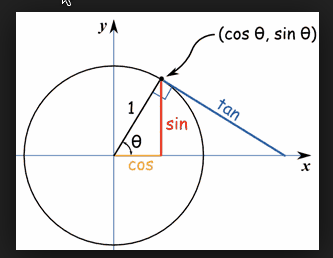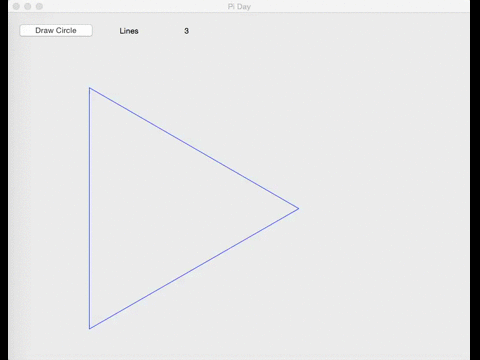Pi day this year is special because the year also fits into Pi, making it possible to have a specific “pi-time” (at least in the US where we write the month first). March 14, 2015 at 9:26:54AM has this value of Pi: 3.141592654.

In honor of Pi Day, I’ve created a small Xojo app that will draw a circle by using line segments, Pi, Sine and Cosine. The circle is first drawn using only three segments which actually draws a triangle. Four segments draws a square. Five segments starts to show a “circular” shape. As the number of segments increases (up to about 150), the shape becomes more and more circular.

In order to do this, you’ll need to know just a tiny bit of trigonometry so that you can use Sine and Cosine. Essentially, the code calculates a line from a start position to an end position. The end position is calculated by using Cosine to get the X value on the graph. Sine is used to get the Y value on the graph. Here is a graph that might help explain it better:The code loops through the circumference of the circle by the line segment length.

If you run the project, you can watch the circle get better as the number of steps increases. The Pi Day project is part of the Examples that are included with Xojo: Examples/Misc/PiDay